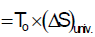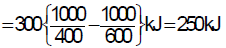Courses

# Availability, Irreversibility, Thermodynamic Relations - MCQ Test 1

## 20 Questions MCQ Test Mock Test Series - Mechanical Engineering (ME) for GATE 2020 | Availability, Irreversibility, Thermodynamic Relations - MCQ Test 1

Description
This mock test of Availability, Irreversibility, Thermodynamic Relations - MCQ Test 1 for Mechanical Engineering helps you for every Mechanical Engineering entrance exam. This contains 20 Multiple Choice Questions for Mechanical Engineering Availability, Irreversibility, Thermodynamic Relations - MCQ Test 1 (mcq) to study with solutions a complete question bank. The solved questions answers in this Availability, Irreversibility, Thermodynamic Relations - MCQ Test 1 quiz give you a good mix of easy questions and tough questions. Mechanical Engineering students definitely take this Availability, Irreversibility, Thermodynamic Relations - MCQ Test 1 exercise for a better result in the exam. You can find other Availability, Irreversibility, Thermodynamic Relations - MCQ Test 1 extra questions, long questions & short questions for Mechanical Engineering on EduRev as well by searching above.
QUESTION: 1

### A steel billet of 2000 kg mass is to be cooled from 1250 K to 450 K. The heat released during this process is to be used as a source of energy. The ambient temperature is 303 K and specific heat of steel is 0.5 kJ/kg K. The available energy of this billet is:

Solution: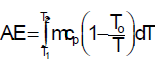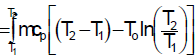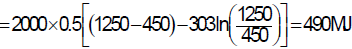QUESTION: 2

### A heat reservoir at 900 K is brought into contact with the ambient at 300 K for a short time. During this period 9000 kJ of heat is lost by the heat reservoir. The total loss in availability due to this process is:

Solution:

The availability of a thermal reservoir is equivalent to the work output of a
Carnot heat engine operating between the reservoir and the environment. Here as there is no change in the temperatures of source (reservoir) or the sink (atmosphere), the initial and final availabilities are same. Hence, there is no loss in availability.

QUESTION: 3

### What will be the loss of available energy associated with the transfer of 1000 kJ of heat from constant temperature system at 600 K to another at 400 K when the environment temperature is 300 K?

Solution:

Loss of available energy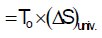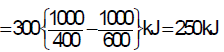QUESTION: 4

A control mass gives out 10 kJ of energy in the form of heat transfer at 500°C. Find the change in availability of the control mass.

Solution:

Explanation: ∆Φ = -[1-(To/Th)]Q = -[1-(298.15/773.15)](10) = −6.14 kJ.

QUESTION: 5

For a steady flow process from state 1 to 2, enthalpy changes from h1 = 400 kJ/kg to h2 = 100 kJ/kg and entropy changes from s1 = 1.1 kJ/kg-K to s2 = 0.7 kJ/kg-K. Surrounding environmental temperature is 300 K. Neglect changes in kinetic and potential energy. The change in availability of the system is:

Solution: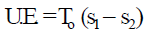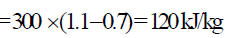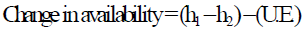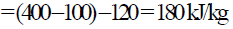QUESTION: 6

Consider the following statements:

Availability is the maximum theoretical work obtainable.

Clapeyron's equation for dry saturated steam is given by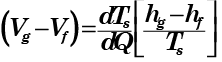A gas can have any temperature at a given pressure unlike a vapour which has a fixed temperature at a given pressure.

Joule Thomson coefficient is expressed as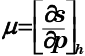Of these statements

Solution:

The availability of a given system is defined as the maximum useful work that can be obtained in a process in which the system comes to equilibrium with the surroundings or attains a dead state. Clearly, the availability of a system depends on the condition of the system as well as those of the surroundings.

QUESTION: 7

Neglecting changes in kinetic energy and potential energy, for unit mass the availability in a non-flow process becomes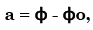where ɸ is the availability function of the

Solution:
QUESTION: 8

The irreversibility is defined as the difference of the maximum useful work and actual work: I = Wmax,useful- Wactual. How can this be alternatively expressed?

Solution: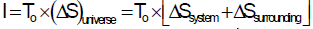QUESTION: 9

Which of the following statement is incorrect?

Solution:
QUESTION: 10

An inventor claims that heat engine has the following specifications:

Power developed = 50 kW; Fuel burned per hour = 3 kg

Heating value of fuel =75,000 kJ per kg; Temperature limits = 627°C and 27°C

Cost of fuel = Rs. 30/kg; Value of power = Rs. 5/kWh

Solution:

Maximum possible efficiency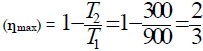Maximum possible Power output with this machine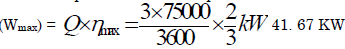So above demand is impossible.

QUESTION: 11

Increase in entropy of a system represents

Solution:
QUESTION: 12

Assertion (A): When a gas is forced steadily through an insulated pipe containing a porous plug, the enthalpy of gas is the same on both sides of the plug.

Reason (R): The gas undergoes an isentropic expansion through the porous plug.

Solution:

Expansion through the porous plug is adiabatic as no heat added or rejected to the system. It is not reversible, due to large irreversibility entropy increases so it is not an isentropic process.

QUESTION: 13

Availability of a system at any given state is:

Solution:

Maximum useful work, i.e. total work minus pdv work. Not maximum work.

QUESTION: 14

Consider the following two processes:

a. A heat source at 1200 K loses 2500 kJ of heat to sink at 800 K

b. A heat source at 800 K loses 2000 kJ of heat to sink at 500 K

Which of the following statements is TRUE?

Solution:
QUESTION: 15

What is the loss of available energy associated with the transfer of 1000 kJ of heat from a constant temperature system at 600 K to another at 400 K when the environmental temperature is 300 K?

Solution: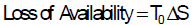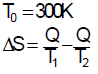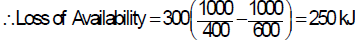QUESTION: 16

Assertion (A): The change in availability of a system is equal to the change in the Gibbs function of the system at constant temperature and pressure.
Reason (R): The Gibbs function is useful when evaluating the availability of systems in which chemical reactions occur.

Solution:
QUESTION: 17

Availability function for a closed system is expressed as:

Solution:
QUESTION: 18

Consider the following statements:

1. Availability is generally conserved

2. Availability can either be negative or positive

3. Availability is the maximum theoretical work obtainable

4. Availability can be destroyed in irreversibility

Of these correct statements are:

Solution:

Availability is the maximum theoretical work obtainable and it can be destroyed in irreversibility.

QUESTION: 19

Assertion (A): All constant entropy processes are adiabatic, but all adiabatic processes are not isentropic.

Reason (R): An adiabatic process which resists the exchange of energy to the surroundings may have irreversibility due to friction and heat conduction.

Solution:

A is false, For a process due to irreversibility entropy will increase and actual process may be 1–2' but due to heat loss to the surroundings, may 2' coincide with 2 but the process not adiabatic. So, all isentropic process is not adiabatic.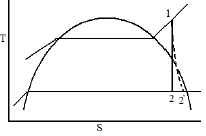QUESTION: 20

What will be the loss of available energy associated with the transfer of 1000 kJ of heat from constant temperature system at 600 K to another at 400 K when the environment temperature is 300 K?

Solution:

Loss of available energy